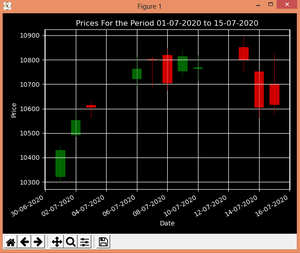# Plot Candlestick Chart using mplfinance module in Python

• Difficulty Level : Easy
• Last Updated : 16 Dec, 2021

Candlestick chart are also known as a Japanese chart. These are widely used for technical analysis in trading as they visualize the price size within a period. They have four points Open, High, Low, Close (OHLC). Candlestick charts can be created in python using a matplotlib module called mplfinance

#### Installation:

`pip install mplfinance`

## mplfinance.candlestick_ohlc()

This function is used to plot Candlestick charts.

Syntax: mplfinance.candlestick_ohlc(ax, quotes, width=0.2, colorup=’k’, colordown=’r’, alpha=1.0)
Parameters:

• ax: An Axes instance to plot to.
• quotes: sequence of (time, open, high, low, close, …) sequences.
• width: Fraction of a day for the rectangle width.
• colorup: The color of the rectangle where close >= open.
• colordown: The color of the rectangle where close < open.
• alpha: (float) The rectangle alpha level.

Returns: returns (lines, patches) where lines are a list of lines added and patches is a list of the rectangle patches added.

To plot the chart, we will take data from NSE for the period 01-07-2020 to 15-07-2020, the data is available for download in a csv file, or can be downloaded from here. For this example, it is saved as ‘data.csv’.
We will use the pandas library to extract the data for plotting from data.csv.

Below is the implementation:

## Python3

 `# import required packages``import` `matplotlib.pyplot as plt``from` `mplfinance ``import` `candlestick_ohlc``import` `pandas as pd``import` `matplotlib.dates as mpdates` `plt.style.use(``'dark_background'``)` `# extracting Data for plotting``df ``=` `pd.read_csv(``'data.csv'``)``df ``=` `df[[``'Date'``, ``'Open'``, ``'High'``,``         ``'Low'``, ``'Close'``]]` `# convert into datetime object``df[``'Date'``] ``=` `pd.to_datetime(df[``'Date'``])` `# apply map function``df[``'Date'``] ``=` `df[``'Date'``].``map``(mpdates.date2num)` `# creating Subplots``fig, ax ``=` `plt.subplots()` `# plotting the data``candlestick_ohlc(ax, df.values, width ``=` `0.6``,``                 ``colorup ``=` `'green'``, colordown ``=` `'red'``,``                 ``alpha ``=` `0.8``)` `# allow grid``ax.grid(``True``)` `# Setting labels``ax.set_xlabel(``'Date'``)``ax.set_ylabel(``'Price'``)` `# setting title``plt.title(``'Prices For the Period 01-07-2020 to 15-07-2020'``)` `# Formatting Date``date_format ``=` `mpdates.DateFormatter(``'%d-%m-%Y'``)``ax.xaxis.set_major_formatter(date_format)``fig.autofmt_xdate()` `fig.tight_layout()` `# show the plot``plt.show()`

Output :Candlestick Chart

My Personal Notes arrow_drop_up